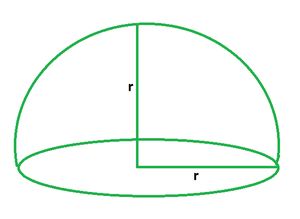# Surface Area of Hemisphere formula

• Last Updated : 01 Jun, 2022

A hemisphere is formed when a plane divides a sphere into two equal parts. To put it another way, a hemisphere is an exact half of a sphere in geometry. It is made up of two parts: hemi, which means half, and sphere, which is a 3D mathematical shape. When a sphere is sliced at the precise center along its diameter, two equal hemispheres are generated. As a result, a hemisphere is a three-dimensional geometric object consisting of half of a sphere with one side flat and the other as a round bowl.### Total Surface Area Formula of Hemisphere

A hemisphere’s total surface area is defined as the total space filled by it. It is given by the sum of its curved surface area and base area. Its formula equals three times the product of pi and the square of the radius of the hemisphere.

TSA = 3πr2

Where,

TSA is the total surface area,

π is a constant with the value of 3.14,

r is the radius of hemisphere.

### Curved Surface Area Formula of Hemisphere

A hemisphere’s curved surface area is defined as the area covered by its curved surface. It is equal to half of the total surface area of a sphere. Its formula equals two times the product of pi and the square of the radius of the hemisphere.

CSA = 2πr2

Where,

CSA is the curved surface area,

π is a constant with the value of 3.14,

r is the radius of hemisphere.

### Sample Problems

Problem 1: Calculate the total surface area of a hemisphere of radius 3 m.

Solution:

We have,

r = 3

Using the formula we get,

TSA = 3πr2

= 3 (3.14) (3)2

= 84.78 sq. m

Problem 2: Calculate the total surface area of a hemisphere of radius 4 m.

Solution:

We have,

r = 4

Using the formula we get,

TSA = 3πr2

= 3 (3.14) (4)2

= 150.72 sq. m

Problem 3: Calculate the radius of a hemisphere if its total surface area is 200 sq. m.

Solution:

We have,

A = 200

Using the formula we get,

A = 3πr2

=> r2 = A/3π

=> r2 = 200/3 (3.14)

=> r = 4.60 m

Problem 4: Calculate the radius of a hemisphere if its total surface area is 350 sq. m.

Solution:

We have,

A = 200

Using the formula we get,

A = 3πr2

=> r2 = A/3π

=> r2 = 350/3 (3.14)

=> r = 6.09 m

Problem 5: Calculate the curved surface area of a hemisphere of radius 5 m.

Solution:

We have,

r = 5

Using the formula we get,

CSA = 2πr2

= 3 (3.14) (5)2

= 235.5 sq. m

Problem 6: Calculate the curved surface area of a hemisphere of radius 4 m.

Solution:

We have,

r = 4

Using the formula we get,

CSA = 2πr2

= 3 (3.14) (4)2

= 150.72 sq. m

Problem 7: Calculate the radius of a hemisphere if its curved surface area is 790 sq. m.

Solution:

We have,

A = 790

Using the formula we get,

A = 2πr2

=> r2 = A/2π

=> r2 = 350/2 (3.14)

=> r = 7.46 m

My Personal Notes arrow_drop_up